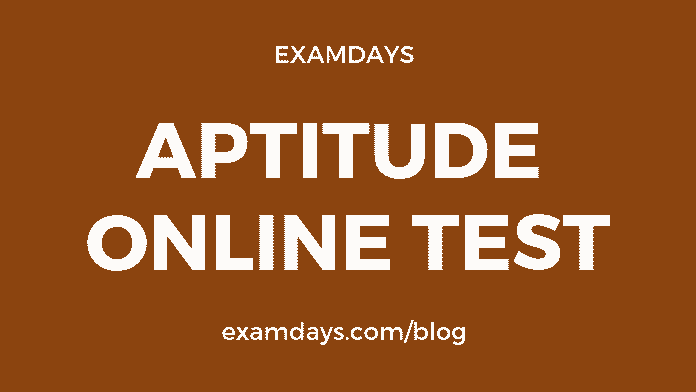# Aptitude Online Test Quiz #9 with Answers

0# Aptitude Online Test

General quantitative aptitude online test is more important for the various examinations, such as SSC, Railways RRB, PSU, State PSC, IBPS Banks, and other Government notifications, The aptitude questions practice make sense in the examination purpose, candidates should practice as many of questions and different models of questions.

Q1. Find the average of all the numbers between 6 and 34 which are divisible by 5?

1. 18
2. 20
3. 24
4. 30

20

Q2. The average age of 40 students is 8 years. If the age of the teacher is also included, then their average age increases by half a year. What is the age of the teacher?

1. 45 years
2. 48.5 years
3. 28.5 years
4. 26.5 years

28.5 years

Q3. The number of employees in the IT department in institute E is approximately what per cent more than that in the Finance department?

1. 47
2. 57
3. 67
4. 77

57

Q4. The number of employees in the IT department of institute B is approximately what per cent of the total number of employees in the same institute?

1. 13
2. 23
3. 33
4. 43

23

Q5. What is the total number of employees in all the departments in institute D?

1. 243
2. 936
3. 543
4. None of these

None of these

Q6. A train passes a telegraph post in 8 seconds and a 264 m long bridge in 20 seconds. What is the length of the train?

1. 180 m
2. 176 m
3. 164 m
4. 158 m

176 m

Q7. There are 2 trains P and Q moving in the same direction. They are of equal length and cross a stationary pole in 5 seconds and 6 seconds respectively. In how much time would they cross each other?

1. 30 seconds
2. 11 seconds
3. 60 seconds
4. 1 Second

60 seconds

Q8. A train having length 150 m passes a platform of 550 m length. The time taken for it is 56 seconds. In how much time will this train take to pass the platform of 250 m length?

1. 32 seconds
2. 24 seconds
3. 28 seconds
4. 30 seconds

32 seconds

Q9. ¾ part of the tank is full of water. When 30 litres of water is taken out it becomes empty. The capacity of the tank is?

1. 36 litres
2. 42 litres
3. 40 litres
4. 38 litres

40 litres

Q10.  When a number is divided by 893 the remainder is 193. What will be the remainder when it is divided by 47?

1. 19
2. 5
3. 33
4. 23